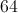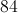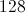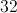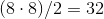# High School Math : How to find the area of a 45/45/90 right isosceles triangle

## Example Questions

### Example Question #1 : How To Find The Area Of A 45/45/90 Right Isosceles Triangle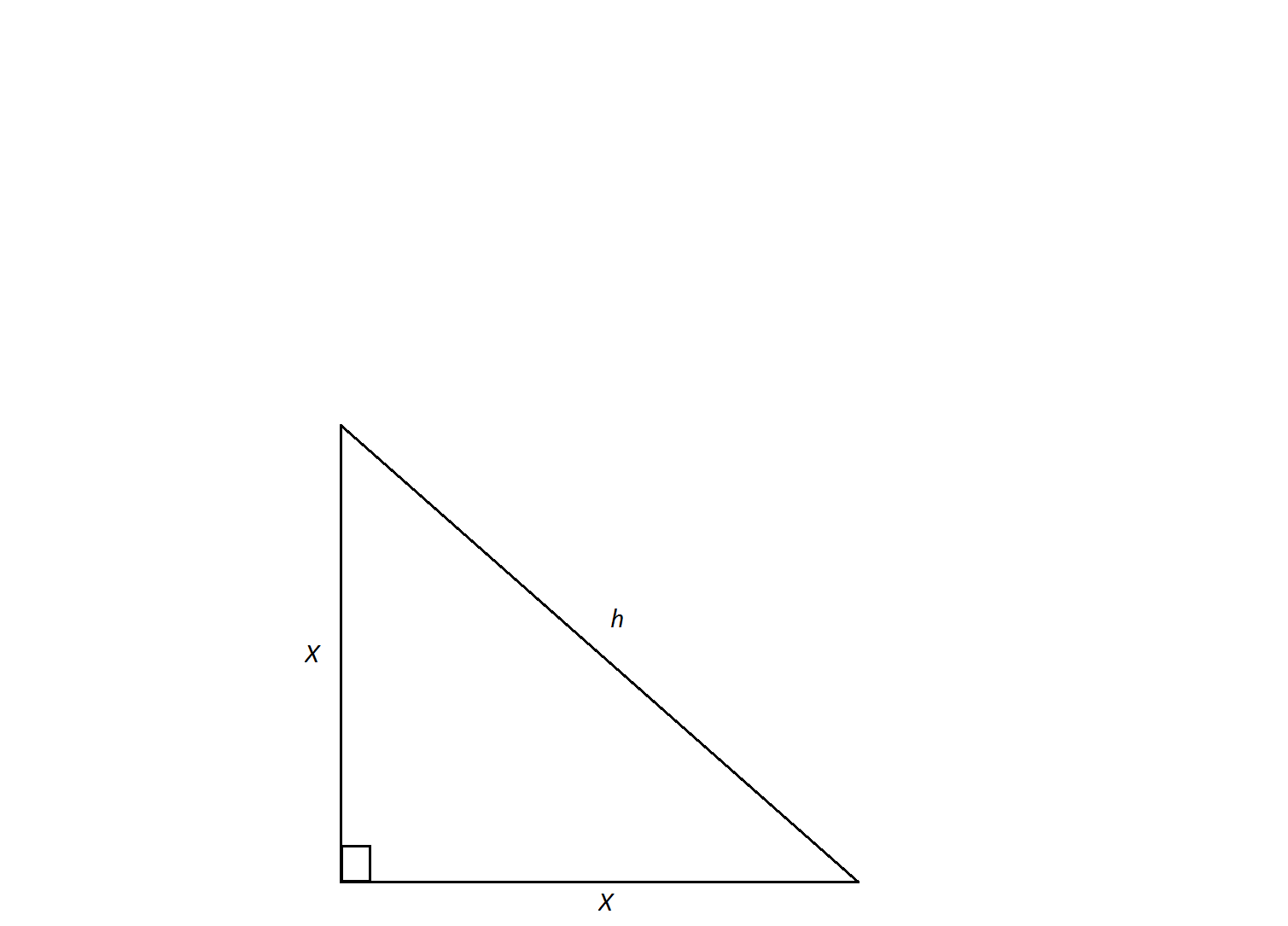An isosceles right triangle has a hypotenuse of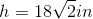.  Find its area.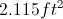Not enough information to solve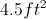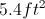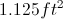Explanation:

In order to calculate the triangle's area, we need to find the lengths of its legs.  An isosceles triangle is a special triangle due to the values of its angles. These triangles are referred to as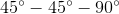triangles and their side lengths follow a specific pattern that states that one can calculate the length of the legs of an isoceles triangle by dividing the length of the hypotenuse by the square root of 2.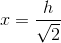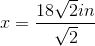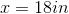Now we can calculate the area using the formula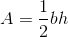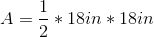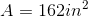Now, convert to feet.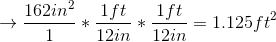### Example Question #2 : How To Find The Area Of A 45/45/90 Right Isosceles Triangle

The base of a right isosceles triangle is 8 inches.  The hypotenuse is not the base.  What is the area of the triangle in inches?